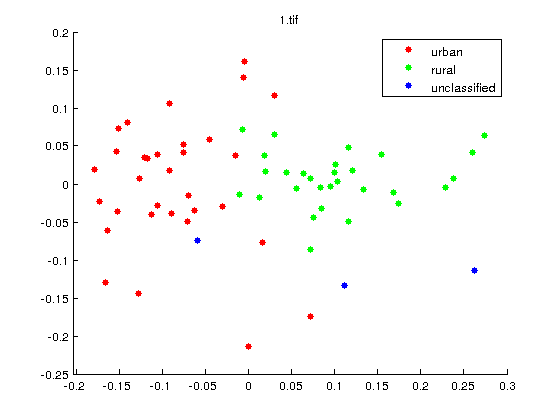/  Top Machine learning interview questions and answers   /  What do you mean by Multi-Dimensional Scaling (MDS)?## What do you mean by Multi-Dimensional Scaling (MDS)?

Multidimensional scaling is a visual representation of distances or dissimilarities between sets of objects. “Objects” can be colors, faces, map coordinates. MDS does finds set of vectors in p-dimensional space such that the matrix of Euclidean distances among them corresponds as closely as possible to some function of the input matrix according to a criterion function called stress.

The input to multidimensional scaling is a distance matrix. The output is typically a two-dimensional scatterplot, where each of the objects is represented as a point.